### Home > PC > Chapter 6 > Lesson 6.4.2 > Problem6-157

6-157.
1. Solve for x. Homework Help ✎

1. 2x2 − 5 = 2x

2. 6x4 = x2 + 5

3.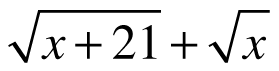= 7

4. 2 +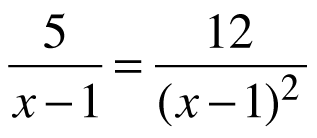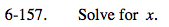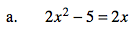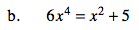1. Bring all terms to the left side and set = 0.
2. Factor.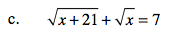$\text{1. Subtract the }\sqrt{x}\text{ from both sides.}$

2. Then square both sides and multiply out the right side.

x = 4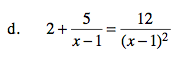Multiply all terms by (x − 1)2.

$x=3,\frac{5}{2}$